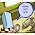## Friday, January 30, 2009

### When to declare a problem is hard?/Constructive versions of Dilworth's theorem

Why do we think solving P vs NP is hard? There are some matematical reasons (oracles, Natural Proofs, Algebraization) but there is also this notion that many smart peoplet of people have worked on it.

If there is an open problem that not that many people have worked on, even if they are brilliant people, its hard to know how hard it is. I present an open problem of this nature.

Recall Dilworths theorem: If P is a partial order of width w (so the largest set of inc elements has w elements) then there exists w chains that cover P (there are w disjoint linear suborders of P that contain all of P). Dilworths theorem: If P is a partial order of width w (so the largest set of inc elements has w elements) then there exists w chains that cover P (there are w disjoint linear suborders of P that contain all of P). Dilworths theorem: If P is a partial order of width w (so the largest set of inc elements has w elements) then there exists w chains that cover P (there are w disjoint linear suborders of P that contain all of P).

Dilworths theorem is usually proven for the finite case and then, by the usual compactness arguments, holds for the infinite case. So the infinite case has a non-effective proof. The recursive math program tells us to ask the following questions.
1. Is there a computable function that will, given the Turing machine for a partial order on the set N (input (x,y), output 1 if x&le y, 2 if y &le x, 3 if x and y are incomparable) and given the width w, output a set of w total Turing machines that decide w disjoint chains that cover the partial order.
2. If not (and the answer is NO) then is there a weaker result that is effectively true?
Here is what is known (roughly):
1. For every w there is a computable partial order of width w that cannot be covered with ((w+1) choose 2)-1 recursive chains (it can be covered with ((w+1) choose 2) recursive chains). Proven by Szemeredi and Trotter but reported in Recursive Ordered Sets by Henry A. Kierstead, In the book Combinatorics and Ordered Sets. American Mathematical Society, 1986. The proof can also be found in my survey of recursive combinatorics.
2. There is an algorithm that will, given a computable partial order of width w, find a (5w-1)/4 computable chains that cover it. (An effective version of {D}ilworth's Theorem by Henry A. Kierstead. Transactions of the American Math Society, vol 268, 63--77, 1981.)
The open problem is to CLOSE THAT GAP! How hard is this? I've asked around and it seems that while Hal Kierstead is a brilliant guy and he's had a few brilliant people have working on it, it remains uncracked. Does this mean its hard? Not necc. No matter how Brilliant the people working on it are, I think you need to have a community of people working on a problem for a number of years before you can say WOW, thats a hard problem!.

1.2.The theorem you quote as desired, gets w as is with no computable justification.

It seems to me that to consider a truly computable version, the input should contain a proof that the machine induces a partial order of width w.

Is there a reason my intuition is wrong?

3.Anon2- There are many different ways to fomulate the question
is their a constructive proof of this theorem?''. I presented
one approach- the computability
approach.

rigorous. You would need to specify
the proof system that you allow
proofs that partial orders have
width w to be in.
YES this could be interesting
I do not believe it has been worked on but you should ask
the FOM website (Foundations of
Math).

ALSO of interest- the REVERSE MATH
program which also looks at
the question of how nonconstructive
a theorem is in a different
framework.

bil gasarch

4.The funny thing is, once the idea that a problem is hard is perpetuated sufficiently widely, it attracts fewer and fewer people to work on it, and over time, builds up a reputation of being hard even if not many people have looked at it closely enough.
By association, "similar" problems also become labeled as hard - I am not sure many people have thought about P vs PSPACE or NL vs NP deeply enough. Thus when something like NL = co-NL is proved, it comes as a huge surprise.

5.NL vs co-NL is a beautiful result but by that point in time it was already known that the logspace hierarchy collapsed to the second level in a result due to Lange, Kirsig, and Jenner. Now that result was a surprise.

Lots of people have worked on much problems that would be implied by a separation of NL and NP and are much easier. Uniform circuit lower bounds, time-space tradeoff lower bounds, etc, We're stuck at uniform-AC^0 vs NP - the best we have is a separation of this from #P. A large effort in complexity has gone into this problem.

On the flip side there has been much less work on P vs PSPACE.

6.OFF TOPIC: I seem to recall a letter from Kurt Godel (to John von Neumann?) saying something to the effect that Godel was doubtful that the intuitive concept of computation could ever be satisfactorily formalized. If this isn't a false memory, I would appreciate a pointer to the exact quote.

Kirk Pruhs